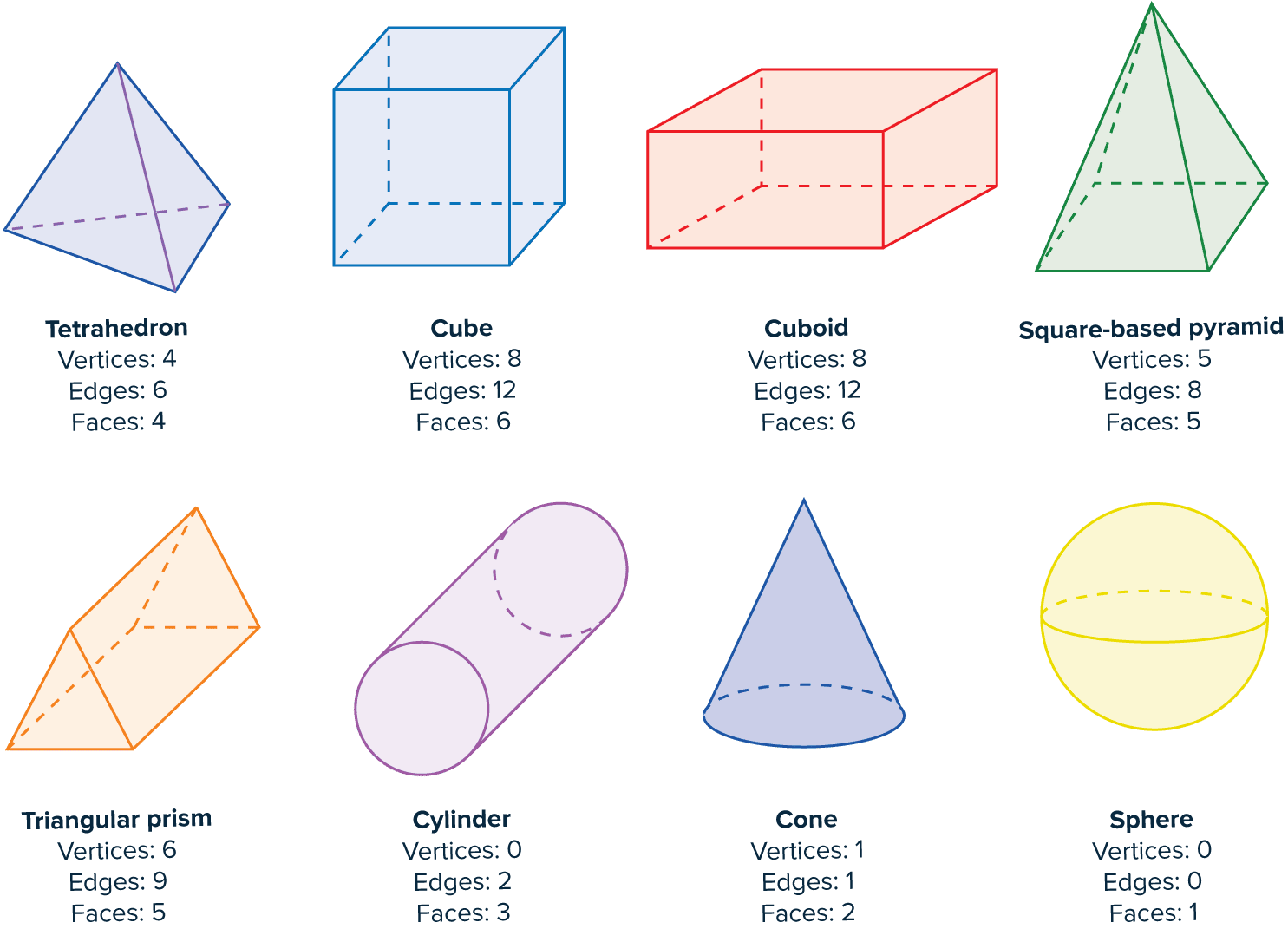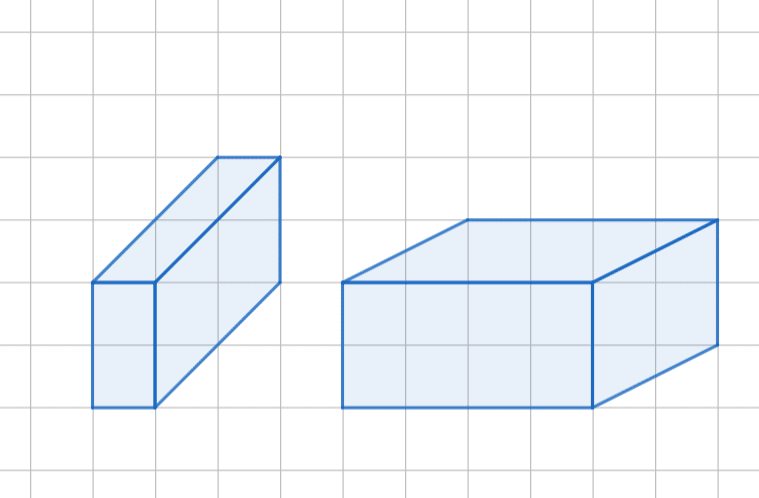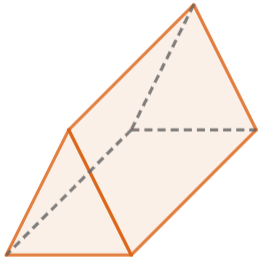# 3D Shapes, Faces, Edges and Vertices

GCSEKS3Level 1-3AQACambridge iGCSEEdexcelEdexcel iGCSEOCRWJEC

## 3D Shapes

3D shapes have a length, width and depth. All objects you can touch in the real word are technically 3D. Some 3D shapes you’ll see much more frequently than others, and their names are important! They’re listed further down.

Make sure you are happy with the following topics before continuing:

Level 1-3GCSEKS3AQAEdexcelOCRWJECCambridge iGCSEEdexcel iGCSE

## Vocabulary

A quick note on some vocabulary of 3D shapes:

1. Vertices – a vertex is where two or more edges meet.

2. Edges – the straight and curved lines that form the shape are edges.

3. Faces – the 2D shapes on the outsides of the 3D shapes (e.g. the $6$ squares on the outside of a cube) are called faces.

Some of these shapes you’ll naturally be more familiar with than others.

Note: drawing 3D shapes is hard but something you may be asked to do!

Level 1-3GCSEKS3AQAEdexcelOCRWJECCambridge iGCSEEdexcel iGCSE

## Common 3D Shapes

A quick note on some 3D shapes and their Faces, Edges and Vertices.Note: You may see that a cylinder has only $2$ faces and $1$ curved surface. Likewise, a cone has $1$ face and $1$ curved surface, and a sphere has $0$ faces and $1$ curved surface.

Level 1-3GCSEKS3AQAEdexcelOCRWJECCambridge iGCSEEdexcel iGCSE

## 3D Shapes, Faces, Edges and Vertices Example Questions

A cuboid has all right-angles, and as a result every single face is a rectangle. There can be lots of different kinds of cuboids, and therefore lots of different correct answers to this question. Two examples of cuboids are shown below.Drawing 3D shapes is tricky, as mentioned. If you didn’t get this quite right, have a think about these two cuboids and try to work out what makes them correct.Gold Standard Education

a) This is a square-based pyramid. And don’t forget “square-based”, it’s important! Pyramids can have all kinds of different shaped bases. A tetrahedron might also be called a “triangle-based pyramid”, for example.

*If you said rectangle-based pyramid, because you were unsure whether the base in the picture is a square, this is also accepted.

b) The circular base and single sharp vertex mean: this is a cone.

c) The two identical circular ends mean: this is a cylinder.Gold Standard Education

Firstly, let’s recall what a triangular prism looks like.So, it has two triangular faces – one on the front and one at the back.

Then, we have to consider the faces going around the middle of the shape. Looking, we can see that there are three rectangular faces.

Therefore, the total number of faces on a triangular prism is $2+3=5$.Gold Standard Education

The shape, called a frustum, is a square based pyramid with a portion of the top removed. Hence , it has two square surfaces at the top and bottom as well as four trapezia. It total the shape has 6 faces.

Counting the lines that make up the shape: there is 4 on the top, 4 on the bottom and 4 connecting the bottom to the top. So there are 12 edges altogether.

Vertices are the points where the edges intersect. In this instance  there are 8 vertices.Gold Standard Education

The shape is a sphere which has a single surface hence has only 1 face as well as 0 edges and 0 vertices.Gold Standard Education

## 3D Shapes, Faces, Edges and Vertices Worksheet and Example Questions

### (NEW) 3D Shapes Exam Style Questions - MME

Level 1-3GCSENewOfficial MMEProduct